# Classify Defects on Wafer Maps Using Deep Learning

This example shows how to classify eight types of manufacturing defects on wafer maps using a simple convolutional neural network (CNN).

Wafers are thin disks of semiconducting material, typically silicon, that serve as the foundation for integrated circuits. Each wafer yields several individual circuits (ICs), separated into dies. Automated inspection machines test the performance of ICs on the wafer. The machines produce images, called wafer maps, that indicate which dies perform correctly (pass) and which dies do not meet performance standards (fail).

The spatial pattern of the passing and failing dies on a wafer map can indicate specific issues in the manufacturing process. Deep learning approaches can efficiently classify the defect pattern on a large number of wafers. Therefore, by using deep learning, you can quickly identify manufacturing issues, enabling prompt repair of the manufacturing process and reducing waste.

This example shows how to train a classification network that detects and classifies eight types of manufacturing defect patterns. The example also shows how to evaluate the performance of the network.

This example uses the WM-811K Wafer Defect Map data set  . The data set consists of 811,457 wafer maps images, including 172,950 labeled images. Each image has only three pixel values. The value `0` indicates the background, the value `1` represents correctly behaving dies, and the value `2` represents defective dies. The labeled images have one of nine labels based on the spatial pattern of the defective dies. The size of the data set is 3.5 GB.

Set `dataDir` as the desired location of the data set. Download the data set using the `downloadWaferMapData` helper function. This function is attached to the example as a supporting file.

```dataDir = fullfile(tempdir,"WaferDefects"); downloadWaferMapData(dataDir)```

### Preprocess and Augment Data

The data is stored in a MAT file as an array of structures. Load the data set into the workspace.

```dataMatFile = fullfile(dataDir,"MIR-WM811K","MATLAB","WM811K.mat"); waferData = load(dataMatFile); waferData = waferData.data;```

Explore the data by displaying the first element of the structure. The `waferMap` field contains the image data. The `failureType` field contains the label of the defect.

`disp(waferData(1))`
``` waferMap: [45×48 uint8] dieSize: 1683 lotName: 'lot1' waferIndex: 1 trainTestLabel: 'Training' failureType: 'none' ```

#### Reformat Data

This example uses only labeled images. Remove the unlabeled images from the structure.

```unlabeledImages = zeros(size(waferData),"logical"); for idx = 1:size(unlabeledImages,1) unlabeledImages(idx) = isempty(waferData(idx).trainTestLabel); end waferData(unlabeledImages) = [];```

The `dieSize`, `lotName`, and `waferIndex` fields are not relevant to the classification of the images. The example partitions data into training, validation, and test sets using a different convention than specified by `trainTestLabel` field. Remove these fields from the structure using the `rmfield` function.

```fieldsToRemove = ["dieSize","lotName","waferIndex","trainTestLabel"]; waferData = rmfield(waferData,fieldsToRemove);```

Specify the image classes.

```defectClasses = ["Center","Donut","Edge-Loc","Edge-Ring","Loc","Near-full","Random","Scratch","none"]; numClasses = numel(defectClasses);```

To apply additional preprocessing operations on the data, such as resizing the image to match the network input size or applying random train the network for classification, you can use an augmented image datastore. You cannot create an augmented image datastore from data in a structure, but you can create the datastore from data in a table. Convert the data into a table with two variables:

• `WaferImage` - Wafer defect map images

• `FailureType` - Categorical label for each image

```waferData = struct2table(waferData); waferData.Properties.VariableNames = ["WaferImage","FailureType"]; waferData.FailureType = categorical(waferData.FailureType,defectClasses);```

Display a sample image from each input image class using the `displaySampleWaferMaps` helper function. This function is attached to the example as a supporting file.

`displaySampleWaferMaps(waferData)`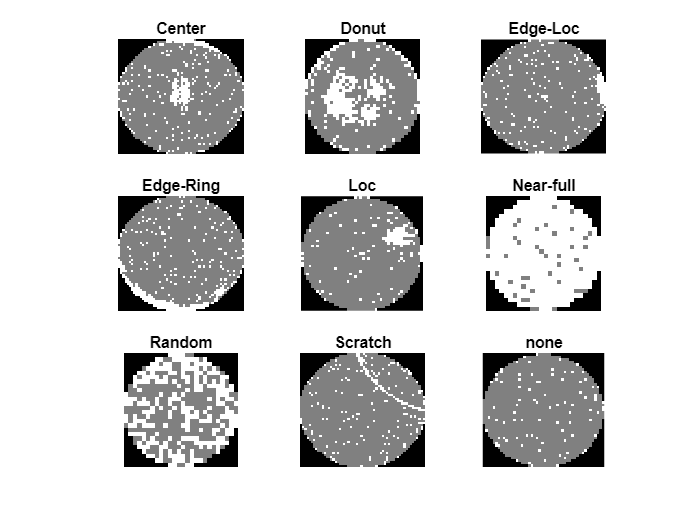#### Balance Data By Oversampling

Display the number of images of each class. The data set is heavily unbalanced, with significantly fewer images of each defect class than the number of images without defects.

`summary(waferData.FailureType)`
``` Center 4294 Donut 555 Edge-Loc 5189 Edge-Ring 9680 Loc 3593 Near-full 149 Random 866 Scratch 1193 none 147431 ```

To improve the class balancing, oversample the defect classes using the `oversampleWaferDefectClasses` helper function. This function is attached to the example as a supporting file. The helper function appends the data set with five modified copies of each defect image. Each copy has one of these modifications: horizontal reflection, vertical reflection, or rotation by a multiple of 90 degrees.

`waferData = oversampleWaferDefectClasses(waferData);`

Display the number of images of each class after class balancing.

`summary(waferData.FailureType)`
``` Center 25764 Donut 3330 Edge-Loc 31134 Edge-Ring 58080 Loc 21558 Near-full 894 Random 5196 Scratch 7158 none 147431 ```

#### Partition Data into Training, Validation, and Test Sets

Split the oversampled data set into training, validation, and test sets using the `splitlabels` (Computer Vision Toolbox) function. Approximately 90% of the data is used for training, 5% is used for validation, and 5% is used for testing.

```labelIdx = splitlabels(waferData,[0.9 0.05 0.05],"randomized",TableVariable="FailureType"); trainingData = waferData(labelIdx{1},:); validationData = waferData(labelIdx{2},:); testingData = waferData(labelIdx{3},:);```

#### Augment Training Data

Specify a set of random augmentations to apply to the training data using an `imageDataAugmenter` object. Adding random augmentations to the training images can avoid the network from overfitting to the training data.

`aug = imageDataAugmenter(FillValue=0,RandXReflection=true,RandYReflection=true,RandRotation=[0 360]);`

Specify the input size for the network. Create an `augmentedImageDatastore` that reads the training data, resizes the data to the network input size, and applies random augmentations.

```inputSize = [48 48]; dsTrain = augmentedImageDatastore(inputSize,trainingData,"FailureType",DataAugmentation=aug);```

Create datastores that read validation and test data and resize the data to the network input size. You do not need to apply random augmentations to validation or test data.

```dsVal = augmentedImageDatastore(inputSize,validationData,"FailureType"); dsVal.MiniBatchSize = 64; dsTest = augmentedImageDatastore(inputSize,testingData,"FailureType");```

### Create Network

Define the convolutional neural network architecture. The range of the image input layer reflects the fact that the wafer maps have only three levels.

```layers = [ imageInputLayer([inputSize 1], ... Normalization="rescale-zero-one",Min=0,Max=2); convolution2dLayer(3,8,Padding="same") batchNormalizationLayer reluLayer maxPooling2dLayer(2,Stride=2) convolution2dLayer(3,16,Padding="same") batchNormalizationLayer reluLayer maxPooling2dLayer(2,Stride=2) convolution2dLayer(3,32,Padding="same") batchNormalizationLayer reluLayer maxPooling2dLayer(2,Stride=2) convolution2dLayer(3,64,Padding="same") batchNormalizationLayer reluLayer dropoutLayer fullyConnectedLayer(numClasses) softmaxLayer classificationLayer];```

### Specify Training Options

Specify the training options for Adam optimization. Train the network for 30 epochs.

```options = trainingOptions("adam", ... ResetInputNormalization=true, ... MaxEpochs=30, ... InitialLearnRate=0.001, ... L2Regularization=0.001, ... MiniBatchSize=64, ... Shuffle="every-epoch", ... Verbose=false, ... Plots="training-progress", ... ValidationData=dsVal, ... ValidationFrequency=20);```

By default, the example loads a pretrained wafer defect classification network. The pretrained network enables you to run the entire example without waiting for training to complete.

To train the network, set the `doTraining` variable in the following code to `true`. Train the model using the `trainNetwork` function.

Train on a GPU if one is available. Using a GPU requires Parallel Computing Toolbox™ and a CUDA® enabled NVIDIA® GPU. For more information, see GPU Computing Requirements (Parallel Computing Toolbox).

```doTraining =false; if doTraining trainedNet = trainNetwork(dsTrain,layers,options); modelDateTime = string(datetime("now",Format="yyyy-MM-dd-HH-mm-ss")); save(fullfile(dataDir,"trained-WM811K-"+modelDateTime+".mat"),"trainedNet"); else downloadTrainedWaferNet(dataDir); trainedNet = load(fullfile(dataDir,"CNN-WM811K.mat")); trainedNet = trainedNet.preTrainedNetwork; end```

### Quantify Network Performance on Test Data

Classify each of test image using the `classify` function.

`defectPredicted = classify(trainedNet,dsTest);`

Calculate the performance of the network compared to the ground truth classifications as a confusion matrix using the `confusionmat` function. Visualize the confusion matrix using the `confusionchart` function. The values across the diagonal of this matrix indicate correct classifications. The confusion matrix for a perfect classifier has values only on the diagonal.

```defectTruth = testingData.FailureType; cmTest = confusionmat(defectTruth,defectPredicted); figure confusionchart(cmTest,categories(defectTruth),Normalization="row-normalized", ... Title="Test Data Confusion Matrix");```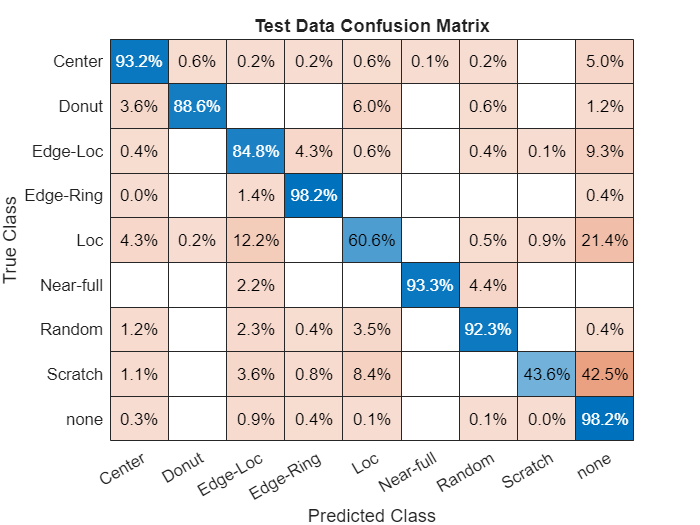#### Precision, Recall, and F1 Scores

This example evaluates the network performance using several metrics: precision, recall, and F1 scores. These metrics are defined for a binary classification. To overcome the limitation for this multiclass problem, you can consider the prediction as a set of binary classifications, one for each class.

Precision is the proportion of images that are correctly predicted to belong to a class. Given the count of true positive (TP) and false positive (FP) classifications, you can calculate precision as:

`$\mathrm{precision}=\frac{\mathrm{TP}\text{\hspace{0.17em}}}{\left(\mathrm{TP}+\mathrm{FP}\right)}$`

Recall is the proportion of images belonging to a specific class that were predicted to belong the class. Given the count of TP and false negative (FN) classifications, you can calculate recall as:

`$\mathrm{recall}=\frac{\mathrm{TP}\text{\hspace{0.17em}}}{\left(\mathrm{TP}+\mathrm{FN}\right)}$`

F1 scores are the harmonic mean of the precision and recall values:

`$\mathrm{F1}=\frac{2*\mathrm{precision}*\mathrm{recall}\text{\hspace{0.17em}}}{\left(\mathrm{precision}+\mathrm{recall}\right)}$`

For each class, calculate the precision, recall, and F1 score using the counts of TP, FP, and FN results available in the confusion matrix.

```prTable = table(Size=[numClasses 3],VariableTypes=["cell","cell","double"], ... VariableNames=["Recall","Precision","F1"],RowNames=defectClasses); for idx = 1:numClasses numTP = cmTest(idx,idx); numFP = sum(cmTest(:,idx)) - numTP; numFN = sum(cmTest(idx,:),2) - numTP; precision = numTP / (numTP + numFP); recall = numTP / (numTP + numFN); defectClass = defectClasses(idx); prTable.Recall{defectClass} = recall; prTable.Precision{defectClass} = precision; prTable.F1(defectClass) = 2*precision*recall/(precision + recall); end```

Display the metrics for each class. Scores closer to 1 indicate better network performance.

`prTable`
```prTable=9×3 table Recall Precision F1 __________ __________ _______ Center {[0.9169]} {[0.9578]} 0.93693 Donut {[0.8193]} {[0.9067]} 0.86076 Edge-Loc {[0.7900]} {[0.8384]} 0.81349 Edge-Ring {[0.9859]} {[0.9060]} 0.94426 Loc {[0.6642]} {[0.8775]} 0.75607 Near-full {[0.7556]} {[ 1]} 0.86076 Random {[0.9692]} {[0.7683]} 0.85714 Scratch {[0.4609]} {[0.8639]} 0.60109 none {[0.9696]} {[0.9345]} 0.95173 ```

#### Precision-Recall Curves and Area-Under-Curve (AUC)

In addition to returning a classification of each test image, the network can also predict the probability that a test image is each of the defect classes. In this case, precision-recall curves provide an alternative way to evaluate the network performance.

To calculate precision-recall curves, start by performing a binary classification for each defect class by comparing the probability against an arbitrary threshold. When the probability exceeds the threshold, you can assign the image to the target class. The choice of threshold impacts the number of TP, FP, and FN results and the precision and recall scores. To evaluate the network performance, you must consider the performance at a range of thresholds. Precision-recall curves plot the tradeoff between precision and recall values as you adjust the threshold for the binary classification. The AUC metric summarizes the precision-recall curve for a class as a single number in the range [0, 1], where 1 indicates a perfect classification regardless of threshold.

Calculate the probability that each test image belongs to each of the defect classes using the `predict` function.

`defectProbabilities = predict(trainedNet,dsTest);`

Use the `rocmetrics` function to calculate the precision, recall, and AUC for each class over a range of thresholds. Plot the precision-recall curves.

```roc = rocmetrics(defectTruth,defectProbabilities,defectClasses,AdditionalMetrics="prec"); figure plot(roc,XAxisMetric="reca",YAxisMetric="prec"); xlabel("Recall") ylabel("Precision") grid on title("Precision-Recall Curves for All Classes")```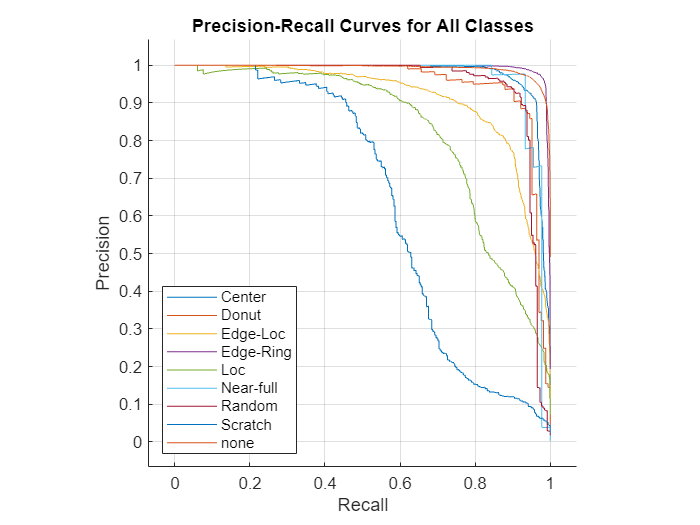The precision-recall curve for an ideal classifier passes through the point (1, 1). The classes that have precision-recall curves that tend towards (1, 1), such as `Edge-Ring` and `Center`, are the classes for which the network has the best performance. The network has the worst performance for the `Scratch` class.

Compute and display the AUC values of the precision/recall curves for each class.

```prAUC = zeros(numClasses, 1); for idx = 1:numClasses defectClass = defectClasses(idx); currClassIdx = strcmpi(roc.Metrics.ClassName, defectClass); reca = roc.Metrics.TruePositiveRate(currClassIdx); prec = roc.Metrics.PositivePredictiveValue(currClassIdx); prAUC(idx) = trapz(reca(2:end),prec(2:end)); % prec(1) is always NaN end prTable.AUC = prAUC; prTable```
```prTable=9×4 table Recall Precision F1 AUC __________ __________ _______ _______ Center {[0.9169]} {[0.9578]} 0.93693 0.97314 Donut {[0.8193]} {[0.9067]} 0.86076 0.89514 Edge-Loc {[0.7900]} {[0.8384]} 0.81349 0.88453 Edge-Ring {[0.9859]} {[0.9060]} 0.94426 0.73498 Loc {[0.6642]} {[0.8775]} 0.75607 0.82643 Near-full {[0.7556]} {[ 1]} 0.86076 0.79863 Random {[0.9692]} {[0.7683]} 0.85714 0.95798 Scratch {[0.4609]} {[0.8639]} 0.60109 0.65661 none {[0.9696]} {[0.9345]} 0.95173 0.99031 ```

### Visualize Network Decisions Using GradCAM

Gradient-weighted class activation mapping (Grad-CAM) produces a visual explanation of decisions made by the network. You can use the `gradCAM` function to identify parts of the image that most influenced the network prediction.

#### `Donut` Defect Class

The `Donut` defect is characterized by an image having defective pixels clustered in a concentric circle around the center of the die. Most images of the `Donut` defect class do not have defective pixels around the edge of the die.

These two images both show data with the `Donut` defect. The network correctly classified the image on the left as a `Donut` defect. The network misclassified the image on the right as an `Edge-Ring` defect. The images have a color overlay that corresponds to the output of the `gradCAM` function. The regions of the image that most influenced the network classification appear with bright colors on the overlay. For the image classified as an `Edge-Ring` defect, the defects at the boundary at the die were treated as important. A possible reason for this could be there are far more `Edge-Ring` images in the training set as compared to `Donut` images.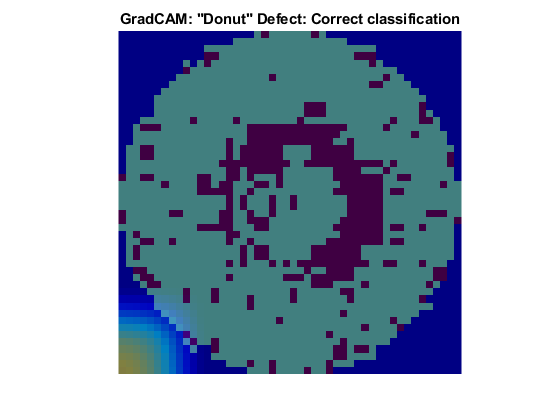#### `Loc` Defect Class

The `Loc` defect is characterized by an image having defective pixels clustered in a blob away from the edges of the die. These two images both show data with the Loc defect. The network correctly classified the image on the left as a `Loc` defect. The network misclassified the image on the right and classified the defect as an `Edge-Loc` defect. For the image classified as an `Edge-Loc` defect, the defects at the boundary at the die are most influential in the network prediction. The `Edge-Loc` defect differs from the `Loc` defect primarily in the location of the cluster of defects.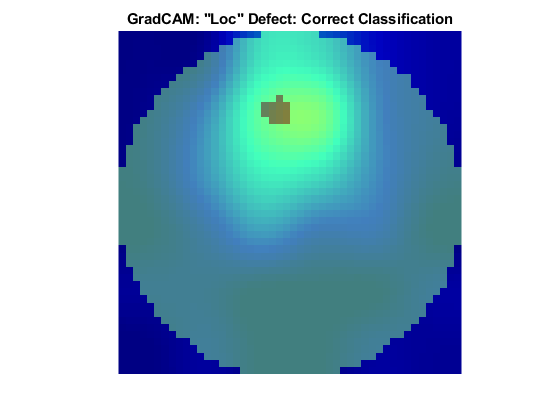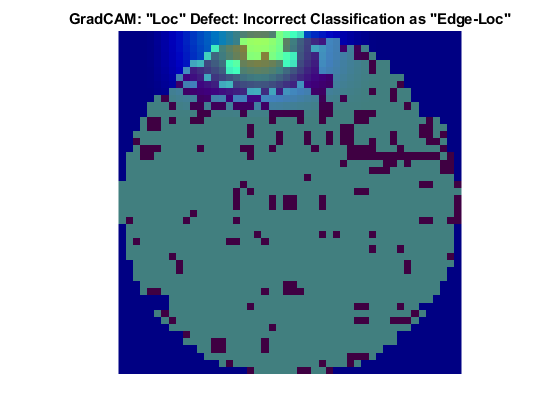#### Compare Correct Classifications and Misclassifications

You can explore other instances of correctly classified and misclassified images. Specify a class to evaluate.

`defectClass =defectClasses(2);`

Find the index of all images with the specified defect type as the ground truth or predicted label.

```idxTrue = find(testingData.FailureType == defectClass); idxPred = find(defectPredicted == defectClass);```

Find the indices of correctly classified images. Then, select one of the images to evaluate. By default, this example evaluates the first correctly classified image.

```idxCorrect = intersect(idxTrue,idxPred); idxToEvaluateCorrect =1; imCorrect = testingData.WaferImage{idxCorrect(idxToEvaluateCorrect)};```

Find the indices of misclassified images. Then, select one of the images to evaluate and get the predicted class of that image. By default, this example evaluates the first misclassified image.

```idxIncorrect = setdiff(idxTrue,idxPred); idxToEvaluateIncorrect =1; imIncorrect = testingData.WaferImage{idxIncorrect(idxToEvaluateIncorrect)}; labelIncorrect = defectPredicted(idxIncorrect(idxToEvaluateIncorrect));```

Resize the test images to match the input size of the network.

```imCorrect = imresize(imCorrect,inputSize); imIncorrect = imresize(imIncorrect,inputSize);```

Generate the score maps using the `gradCAM` function.

```scoreCorrect = gradCAM(trainedNet,imCorrect,defectClass); scoreIncorrect = gradCAM(trainedNet,imIncorrect,labelIncorrect);```

Display the score maps over the original wafer maps using the `displayWaferScoreMap` helper function. This function is attached to the example as a supporting file.

```figure tiledlayout(1,2) t = nexttile; displayWaferScoreMap(imCorrect,scoreCorrect,t) title("Correct Classification ("+defectClass+")") t = nexttile; displayWaferScoreMap(imIncorrect,scoreIncorrect,t) title("Misclassification ("+string(labelIncorrect)+")")```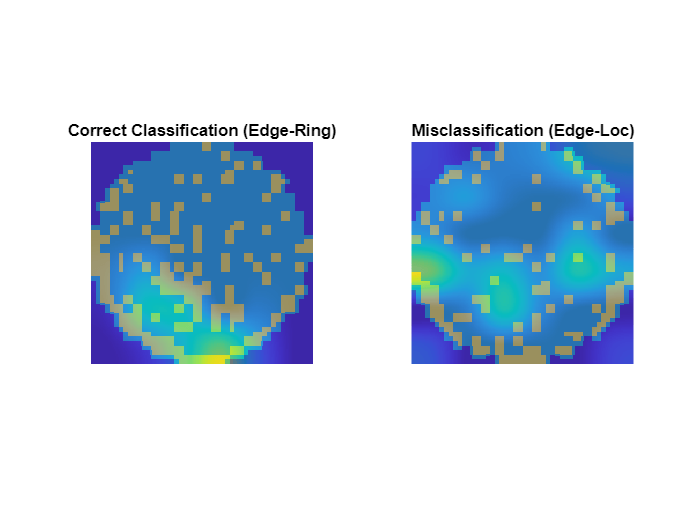### References

 Wu, Ming-Ju, Jyh-Shing R. Jang, and Jui-Long Chen. “Wafer Map Failure Pattern Recognition and Similarity Ranking for Large-Scale Data Sets.” IEEE Transactions on Semiconductor Manufacturing 28, no. 1 (February 2015): 1–12. https://doi.org/10.1109/TSM.2014.2364237.

 Jang, Roger. "MIR Corpora." http://mirlab.org/dataset/public/.

 Selvaraju, Ramprasaath R., Michael Cogswell, Abhishek Das, Ramakrishna Vedantam, Devi Parikh, and Dhruv Batra. “Grad-CAM: Visual Explanations from Deep Networks via Gradient-Based Localization.” In 2017 IEEE International Conference on Computer Vision (ICCV), 618–26. Venice: IEEE, 2017. https://doi.org/10.1109/ICCV.2017.74.

 T., Bex. “Comprehensive Guide on Multiclass Classification Metrics.” October 14, 2021. https://towardsdatascience.com/comprehensive-guide-on-multiclass-classification-metrics-af94cfb83fbd.